# Go Math Grade 3 Chapter 5 Answer Key Pdf Use Multiplication Facts

Go Math Grade 3 Chapter 5 Answer Key Pdf: Get Free pdf of Go Math Grade 3 Answer Key Chapter 5 Use Multiplication Facts here. Solving Go Math Grade 3 Answer Key Chapter 5 helps you to improve your math skills and interest on the subject. This chapter contains all the basic concepts of multiplication facts. If you solve all the questions here then you can become a master in mathematics. Because multiplications and additions are the most essential for scoring the highest marks in the exams.

## Use Multiplication Facts Go Math Grade 3 Chapter 5 Answer Key Pdf

Students can get point to point explanation of the problems by the Math experts. So, you can start your preparation and score good marks in your exams. Check out the overview of all the topics covered in this chapter. Parents can go through the in-depth explanations given in the Grade 3 HMH Go Math Answer Key Chapter 5 Use Multiplication Facts and clarify the doubts of your children. Practice all the sums given in the lessons and try to solve the problems in the review/test. Tap on the below-attached Go Math 3rd standard Grade 3 Chapter Key links and start your preparation.

Lesson 1: Algebra • Describe Patterns

Lesson 2: Algebra • Find Unknown Numbers

Mid-Chapter Checkpoint

Lesson 3: Problem Solving • Use the Distributive Property

Lesson 4: Multiplication Strategies with Multiples of 10

Lesson 5: Multiply 1-Digit Numbers by Multiplies of 10

Chapter 5 Review/Test

### Describe Patterns Page No 265

Describe a pattern for the table. Then complete the table.

Question 1.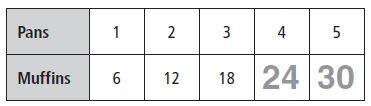Answer: Add 6 muffins for each pan; Multiply the number of pans by 6.

Explanation:

Now we have to multiple no. of pans by 6
4 × 6 = 24; 5 × 6 = 30

Question 2.

 Wagons 2 3 4 5 6 Wheels 8 12 16 _______ _______

 Wagons 2 3 4 5 6 Wheels 8 12 16 20 24

Explanation:

Add 4 wheels for each Wagons; Multiply the number of Wagons by 4
5 × 4 = 20; 6 × 4 = 24

Question 3.

 Vases Flowers 2 14 3 _______ 4 28 5 _______ 6 42

 Vases Flowers 2 14 3 21 4 28 5 35 6 42

Explanation:

Add 7 flowers for each vase; Multiply the number of flowers by 7
3 × 7 = 21; 5 × 7 = 35

Question 4.

 Spiders Legs 1 8 2 _______ 3 24 4 _______ 5 40

 Spiders Legs 1 8 2 16 3 24 4 32 5 40

Explanation:

Add 8 legs for each spider and then multiply the number of spiders by 8
i.e., 8 × 2 = 16; 8 × 4 = 32

Problem Solving

Question 5.
Caleb buys 5 cartons of yogurt. Each carton has 8 yogurt cups. How many yogurt cups does Caleb buy?
_________ yogurt cups

Explanation:

Given,
Caleb buys 5 cartons of yogurt
Each carton has 8 yogurt cups
To find how many yogurt cups does Caleb buy ‘x’
x = 8 × 5 = 40
Thus the number of yogurt cups does Caleb buy is 40 cups

Question 6.
Libby bought 4 packages of pencils. Each package has 6 pencils. How many pencils did Libby buy?
_________ pencils

Explanation:

Libby bought 4 packages of pencils
Each package has 6 pencils
Number of pencils did Libby buy is x
x × 1= 6 × 4
x = 24
Therefore no. of pencils Libby bought = 24 pencils

### Describe Patterns Lesson Check Page No 266

Question 1.
Which of the following describes a pattern in the table?Options:
a. Multiply by 3.
b. Multiply by 5.

Explanation:

From the above table, we can see that each chair is added by 5 for each table.
So, multiply the number of tables by 5
The correct answer is option B

Question 2.
Which number completes this table?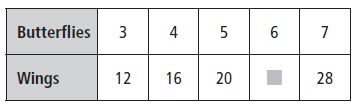Options:
a. 30
b. 20
c. 24
d. 22

Explanation:

Each butterfly is added by 4
Multiply the number of butterflies by 4
4 × 6 = 24 wings
So, the correct answer is option C

Spiral Review

Question 3.
Jennilee buys 7 packs of crayons. There are 6 crayons in each pack. How many crayons does Jennilee buy in all?
Options:
a. 13
b. 36
c. 42
d. 48

Explanation:

Given that, Jennilee buys 7 packs of crayons
There are 6 crayons in each pack
1 pack = 6 crayons
7 packs = x
x × 1 = 6 × 7
x = 42

Question 4.
Maverick has 5 books of circus tickets. Each book has 5 tickets. How many tickets does Maverick have in all?
Options:
a. 10
b. 15
c. 20
d. 25

Explanation:

Maverick has 5 books on circus tickets
Each book has 5 tickets
1 book =5 tickets
5 books = x tickets
x × 1 = 5 × 5
x = 25
Thus the correct answer is option D

Question 5.
Bailey walked his dog 2 times each day for 9 days. How many times did Bailey walk his dog in all?
Options:
a. 9
b. 11
c. 18
d. 27

Explanation:

Given, Bailey walked his dog 2 times each day for 9 days
Number of times did Bailey walk his dog in all = x
x = 9 × 2
x = 18
Thus the correct answer is option C

Question 6.
Drew’s Tree Company delivers pear trees in groups of 4. Yesterday, the company delivered 8 groups of pear trees. How many pear trees were delivered in all?
Options:
a. 12
b. 16
c. 24
d. 32

Explanation:

Given,

Drew’s Tree Company delivers pear trees in groups of 4
Yesterday, the company delivered 8 groups of pear trees
How many pear trees were delivered in all = x
x = 4 × 8
x = 32
Thus the correct answer is option D

### Find Unknown Numbers Page No 271

Find the unknown factor.

Question 1.
n × 3 = 12
Think: How many groups of 3 equal 12?
n = 4

Explanation:

n × 3 = 12
n = 12/4
n = 3

Question 2.
s × 8 = 64
s = ________

Explanation:

How many groups of 8 equals 64?

s × 8 = 64
s = 64/8
s = 8

Question 3.
21 = 7 × n
n = ________

Explanation:

Number of groups 7 equals 21

21 = 7 × n
n = 21/7
n = 3

Question 4.
y × 2 = 18
y = ________

Explanation:

y × 2 = 18
y = 18/2
y = 9

Question 5.
5 × p = 10
p = ________

Explanation:

p is the unknown factor

5p = 10
p = 10/5
p = 2

Question 6.
56 = 8 × t
t = ________

Explanation:

8 × t = 56
8t = 56
t = 56/8
t = 7

Question 7.
m × 4 = 28
m = ________

Explanation:

Here m is the unknown product
4 × m = 28
4m = 28
m = 28/4
m = 7

Question 8.
★ × 1 = 9
★ = ________

Explanation:

Here the symbol ★ is the unknown product
★ = 9/1
★ = 9

Question 9.
18 = 6 × r
r = ________

Explanation:

r is the unknown product
6 × r = 18
r = 18/6
r = 3

Question 10.
u × 5 = 30
u = ________

Explanation:

u is the unknown product
u × 5 = 30
5u = 30
u = 30/5
u = 6

Question 11.
4 × ■ = 24
■ = ________

Explanation:

■ is the unknown product
4 × ■ = 24
■ = 24/4
■ = 6

Question 12.
w × 7 = 35
w = ________

Explanation:

The letter w is the unknown product
w × 7 = 35
w = 35/7
w = 5

Question 13.
b × 6 = 54
b = ________

Explanation:

b × 6 = 54
b = 54/6
b = 9
Thus the unknown product is 9

Question 14.
5 × ▲ = 40
▲ = ________

Explanation:

Here the symbol ▲ is the unknown product
▲ × 5 = 40
▲ = 40/5
▲ = 8

Question 15.
30 = d × 3
d = ________

Explanation:

d is the unknown product
30 = d × 3
30/3 = d
d = 30/3
d = 10

Question 16.
7 × k = 42
k = ________

Explanation:

k is the unknown product
7 × k = 42
k = 42/7
k = 6

Problem Solving

Question 17.
Carmen spent $42 for 6 hats. How much did each hat cost?$ ________

Explanation:

Given that
Carmen spent $42 for 6 hats The cost spent on each hat = x x × 6 = 42 x = 42/6 x = 7 Thus Carmen spent$7 for each hat

Question 18.
Mark has a baking tray with 24 cupcakes. The cupcakes are arranged in 4 equal rows. How many cupcakes are in each row?
________ cupcakes

Explanation:

Mark has a baking tray with 24 cupcakes
The cupcakes are arranged in 4 equal rows
No. of cupcakes in each row = x
x × 4 = 24
x = 24/4
x = 6
Therefore the number of cupcakes in each row = 6

### Find Unknown Numbers Lesson Check Page No 272

Question 1.
What is the unknown factor?
b × 7 = 56
Options:
a. 6
b. 7
c. 8
d. 9

Explanation:

b × 7 = 56
b = 56/7
Now we have to check how many groups of 7 equals 56
7 × 8 = 56
b = 8
Thus the answer is option C

Question 2.
What is the unknown factor shown by this array?3 × ■ = 24
Options:
a. 3
b. 6
c. 8
d. 9

Explanation:

The unknown product is ■
Here we have to find the product of 3 × ■  which equals 24
3 × ■ = 24
■ = 24/3
■ = 8
Therefore the unknown factor shown by this array is 8

Spiral Review

Question 3.
Which is an example of the Commutative Property of Multiplication?
Options:
a. 6 + 4 = 4 + 6
b. 4 × 6 = 6 × 4
c. 4 × 3 = 4 + 8
d. 3 × 6 = 9 × 2

Answer: 4 × 6 = 6 × 4

Explanation:

According to the commutative property of multiplication, changing the order of the numbers we are multiplying, does not change the product.
a × b = b × a
So, the answer is 4 × 6 = 6 × 4

Question 4.
Find the product.
5 × (4 × 2)
Options:
a. 13
b. 22
c. 40
d. 80

Explanation:

This is in the form of a × (b × c)
First, multiply 4 and 2
5 × (4 × 2) = 5 × 8
5 × 8 = 40
Therefore the correct answer is option D

Question 5.
Which number sentence is an example of the Distributive Property?
Options:
a. 4 × 7 = (4 × 3) + (4 × 4)
b. 4 × 7 = 7 × 4
c. 4 × 7 = 28
d. 7 × 4 = 15 + 13

Answer: 4 × 7 = (4 × 3) + (4 × 4)

Example:

To “distribute” means to divide something or give a share or part of something. According to the distributive property, multiplying the sum of two or more addends by a number will give the same result as multiplying each addend individually by the number and then adding the products together.
The example of Distributive Property is 4 × 7 = (4 × 3) + (4 × 4)

Question 6.
In a group of 10 boys, each boy had 2 hats. How many hats did they have in all?
Options:
a. 5
b. 12
c. 20
d. 40

Explanation:

Given that,
Each boy has 2 hats
Group of 10 boys has x hats
x × 1 = 2 × 10
x = 20

### Mid-Chapter Checkpoint Page No 273

Vocabulary
Choose the best term from the box.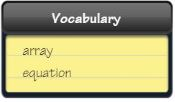Question 1.
An __________ is a number sentence that uses the equal sign to show that two amounts are equal.
__________

Explanation:

The definition of the Equation is the number sentence that uses an equal sign to show that two amounts are equal.

Concepts and Skills

Describe a pattern in the table. Then complete the table.

Question 2.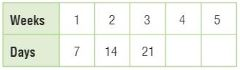Type below:
__________

 Weeks 1 2 3 4 5 Days 7 14 21 28 35

Explanation:

First of all look for the pattern to complete the table.
As you look across the rows you can find the days are increased by 7 for each week.
Now use the pattern to find the number of days in 4 and 5 weeks.
7 × 4 = 28; 7 × 5 = 35

Question 3.Type below:
__________

 Tickets 2 3 4 5 6 Cost $8$12 $16$20 $24 Explanation: Look for the pattern to complete the table. As you look across the rows you can find the cost increased by$4 for each ticket.
Now use the pattern to find the cost for 5 and 6 tickets
4 × 5 = 20; 4 × 6 = 24

Question 4.Type below:
__________

 Project Teams Members 3 9 4 12 5 15 6 18 7 21

Explanation:

Look for the pattern by comparing the columns in the table. You can multiply number of project teams by 3 to find the members.
3 × 5 = 15; 3 × 7 = 21

Question 5.Type below:
__________

 Tables Chairs 1 8 2 16 3 24 4 32 5 40

Explanation:

Look for the pattern by comparing the rows in the table.
Now multiply the number of tables by 8 so that you can find the number of chairs for 3 and 5 tables.
3 × 8 = 24; 5 × 8 = 40

Find the unknown number.

Question 6.
m × 5 = 30
m = _______

Explanation:

m is the unknown product
m × 5 = 30
m = 30/5
m = 6
Therefore the value of m is 6

Question 7.
■ × 6 = 48
■ = _______

Explanation:

■ is the symbol of the unknown product
■ × 6 = 48
■ = 48/6
■ = 8

Question 8.
n = 2 × 10
n = _______

Explanation:

n is the unknown product
We have to product of where 2 and 10 meet
n = 2 × 10
n = 20

Question 9.
4 × 8 = p
p = _______

Explanation:

p is the unknown product
p = 8 × 4
p = 32

Question 10.
25 = y × 5
y = _______

Explanation:

y is the unknown product
y × 5 = 25
y = 25/5
y = 5

Question 11.
★ × 10 = 10
★ = _______

Explanation:

★ is the symbol of the unknown product
★ × 10 = 10
★ = 10/10
★ = 1

### Mid-Chapter Checkpoint Lesson Check Page No 274

Question 12.
Describe a pattern in the table.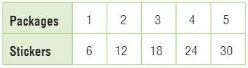Type below:
__________

Explanation:

As you look across the rows, you can see that the number of stickers increases by 6 for each package.

Question 13.
What number makes the equation true?
a × 8 = 72
a = _______

Explanation:

a is the unknown product
Here we have to find the number that makes the equation true
a × 8 = 72
a = 72/8
a = 9

Question 14.
Mia bought 2 copies of the same book. She spent $18. What was the cost of one book?$ _______

Answer: $9 Explanation: Given that, Mia bought 2 copies of the same book. Mia spent$18 for 2 books
Cost of one book = x
x × 2 = $18 x = 18/2 x = 9 Therefore the cost of one book =$9

Question 15.
Kyle saves $10 every week for 6 weeks. How much money will Kyle have in Week 6?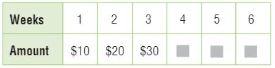a. 60 Answer:  Weeks 1 2 3 4 5 6 Amount$10 $20$30 $40$50 $60 Explanation: Look for the pattern to complete the table. As you look across the rows you can find the amount increased for each week. You can multiply 10 by week 4, 5 and 6 i.e., 10 × 4 = 40; 10 × 5 = 50; 10 × 6 = 60 Question 16. Tennis balls cost$7 for a can of 3. Steve gives the cashier $40 to buy balls and receives$12 in change. How many tennis balls did Steve buy?
_______ tennis balls

Explanation:

Steve spent $40 –$12 = $28 Let y represent the number of cans. Tennis balls cost$7 for a can of 3
7 × y = 28
y = 28/7
y = 4 cans
So, Steve buys 4 cans of 3 tennis balls
Then, we need to multiply the no. of cans, 4, by the number of tennis balls in each can, 3
4 × 3 = 12 tennis balls

### Use the Distributive Property Page No 279

Question 1.
Each time a student turns in a perfect spelling test, Ms. Ricks puts an achievement square on the bulletin board. There are 6 rows of squares on the bulletin board. Each row has 30 squares. How many perfect spelling tests have been turned in?
Think: 6 × 30 = 6 × (10 + 10 + 10)
= 60 + 60 + 60 = 180
180 spelling tests

Explanation:

There are 6 rows of squares on the bulletin board
Each row has 30 squares
We can use the distributive property to find the number of perfect spelling tests have been turned in
6 × 30 = 6 × (10+10+10)
6 × 10 + 6 × 10 + 6 × 10
60 + 60 + 60 = 180 spelling test

Question 2.
Norma practices violin for 50 minutes every day. How many minutes does Norma practice violin in 7 days?
_______ minutes

Explanation:

Given,
Norma practices violin for 50 minutes every day
To find:
How many minutes does Norma practice violin in 7 days?
We can solve this problem by using the distributive property
7 × 50 = 7 × (20 + 30) = (7 × 20) + (7 × 30)
= 350 minutes

Question 3.
A kitchen designer is creating a new backsplash for the wall behind a kitchen sink. The backsplash will have 5 rows of tiles. Each row will have 20 tiles. How many tiles are needed for the entire backsplash?
_______ tiles

Explanation:

Given: The backsplash will have 5 rows of tiles
Each row will have 20 tiles
By using a distributive property we can know the no. of tiles are needed for the entire backsplash
5 × 20 = 5 × (10 + 10)
(5× 10) + (5× 10) = 50 +50
= 100 tiles
Therefore 100 tiles are needed for the entire backsplash

Question 4.
A bowling alley keeps shoes in rows of cubbyholes. There are 9 rows of cubbyholes, with 20 cubbyholes in each row. If there is a pair of shoes in every cubbyhole, how many pairs of shoes are there?
_______ pairs of shoes

Explanation:

There are 9 rows of cubbyholes, with 20 cubbyholes in each row
To find the number of pairs of shoes in every cubbyhole
9 × 20 = 9 × (10 + 10)
9 × 10 + 9 × 10
90 + 90 = 180 pairs of shoes

Question 5.
The third-grade students are traveling to the science museum in 8 buses. There are 40 students on each bus. How many students are going to the museum?
_______ students

Explanation:

Given that, The third-grade students are traveling to the science museum in 8 buses
There are 40 students on each bus
We can know the number of students going to the museum by using the distributive property
8 × 40 = 8 × (20 + 20) = 8 × 20 + 8 × 20
160 + 160 = 320 students
Therefore the number of students going to the museum is 320

### Use the Distributive Property Lesson Check Page No 280

Question 1.
Each snack pack holds 20 crackers. How many crackers in all are there in 4 snack packs?
Options:
a. 60
b. 80
c. 100
d. 800

Explanation:

Given:
Each snack pack holds 20 crackers
To find:
How many crackers in all are there in 4 snack packs
By using the Distributive property we can find the crackers in 4 snack packs
4 × 20 = 4 × (10 + 10)
4 × 10 + 4 × 10 = 40 + 40 = 80
Thus the correct answer is option B

Question 2.
A machine makes 70 springs each hour. How many springs will the machine make in 8 hours?
Options:
a. 500
b. 520
c. 540
d. 560

Explanation:

Given,
A machine makes 70 springs each hour
To find:
How many springs will the machine make in 8 hours
8 × 70 = 8 × (35 + 35)
= (8 × 35) + (8 × 35)
= 280 + 280
= 560
Thus option D is the correct answer

Spiral Review

Question 3.
Lila read 142 pages on Friday and 168 pages on Saturday. Which is the best estimate of how many pages Lila read on Friday and Saturday combined?
Options:
a. 100
b. 200
c. 300
d. 400

Explanation:

Lila read 142 pages on Friday and 168 pages on Saturday
We can estimate the number of pages Lila read on Friday and Saturday combined by using the Distributive property
142 + 168 = (2 × 71) + (2 × 84) = 300
So, the correct answer is option C

Question 4.
Jessica wrote 6 + 6 + 6 + 6 on the board. Which is another way to show 6 + 6 + 6 + 6?
Options:
a. 4 × 4
b. 4 × 6
c. 4 × 4 × 6
d. 6 × 6

Explanation:
Jessica wrote 6 + 6 + 6 + 6 on the board
The another way to write 6 + 6 + 6 + 6 is 4 × 6
Because here 6 is added 4 times. So the multiplication form of 6 + 6 + 6 + 6 is 4 × 6
So, the correct answer is option B

Use the line plot for 5–6.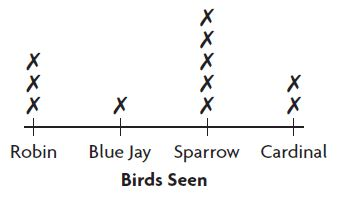Question 5.
Eliot made a line plot to record the number of birds he saw at his bird feeder. How many more sparrows than blue jays did he see?
Options:
a. 2
b. 3
c. 4
d. 5

Explanation:

Number of sparrows = 5
Number of Blue Jays = 1
To know how many more sparrows than blue jays we have to subtract number of blue jay from number of sparrows
= 5 – 1 = 4
So, the correct answer is option C

Question 6.
How many robins and cardinals combined did Eliot see?
Options:
a. 2
b. 3
c. 4
d. 5

Explanation:

Number of robins = 3
Number of Cardinals = 2
Total Number of robins and cardinals = 3 + 2 = 5
So, the answer is option D

### Multiplication Strategies with Multiples of 10 Page No 285

Use a number line to find the product.

Question 1.
2 × 40 = 80Explanation:

The number line given above shows that there are 2 groups of 4 tens
So, 2 × 4 tens
2 × 40 = 80

Question 2.4 × 30 = _______

Explanation:

There are 4 groups of 3 tens
So, the number jumps from 0 to 30, 30 to 60, 60 to 90, and from 90 to 120.
4 × 3 tens = 4 × 30 = 120

Use place value to find the product.

Question 3.
5 × 70 = 5 × _______ tens
= _______ tens = _______

i. 7 tens
ii. 35 tens
iii. 350

Explanation:

70 = 7 × 10 = 7 tens
5 × 70 = 35 × 10 = 35 tens = 350

Question 4.
60 × 4 = _______ tens × 4
= _______ tens = _______

i. 6 tens
ii. 24 tens
iii. 240

Explanation:

Here 60 is multiplied with 4
60 = 6 × 10 = 6 tens
60 × 4 = 6 tens × 4
24 tens = 24 × 10 = 240

Question 5.
7 × 30 = 7 × _______ tens
= _______ tens = _______

i. 3 tens
ii. 21 tens
iii. 210

Explanation:

30 = 3 × 10 = 3 × 1 ten = 3 tens
7 × 30 = 7 × 3 tens
= 21 tens
= 21 × 10 = 210

Question 6.
90 × 3 = _______ tens × 3
= tens = _______

i. 9 tens
ii. 27 tens
iii. 270

Explanation:

90 = 9 × 1 ten = 9 × 10 = 9 tens
9 tens × 3 = 27 tens
27 tens = 27 × 1 ten
= 27 × 10 = 270

Problem Solving

Question 7.
One exhibit at the aquarium has 5 fish tanks. Each fish tank holds 50 gallons of water. How much water do the 5 tanks hold in all?
_______ gallons of water

Explanation:

Given: One exhibit at the aquarium has 5 fish tanks
Each fish tank holds 50 gallons of water
5 × 50 = 5 × 5 tens
= 25 tens = 25 × 1 ten
25 × 10 = 250 gallons of water

Question 8.
In another aquarium display, there are 40 fish in each of 7 large tanks. How many fish are in the display in all?
_______ fish

Explanation:

There are 40 fish in each of 7 large tanks
To know the number of fishe are in the display in all
40 × 7 = 4 × 1 ten = 4 tens
4 tens × 7 = 28 tens
28 × 10 = 280 fishes

### Multiplication Strategies with Multiples of 10 Lesson Check Page No 286

Question 1.
Each bag of pattern blocks contains 50 blocks. To make a class pattern, the teacher combines 4 bags of blocks. How many pattern blocks are there in all?
Options:
a. 20
b. 200
c. 240
d. 250

Explanation:

Given,
Each bag of pattern blocks contains 50 blocks
To make a class pattern, the teacher combines 4 bags of blocks
Here we make use of multiplication strategies to know the number of pattern blocks
50 × 4 = 5 tens × 4
= 20 tens = 20 × 10 = 200
Therefore the correct answer is option B

Question 2.
A deli received 8 blocks of cheese. Each block of cheese weighs 60 ounces. What is the total weight of the cheeses?
Options:
a. 420 ounces
b. 460 ounces
c. 480 ounces
d. 560 ounces

Explanation:

A deli received 8 blocks of cheese
Each block of cheese weighs 60 ounces
60 × 8 = 6 tens × 8
48 tens = 48 × 10 = 480 ounces
So, the correct answer is option C

Spiral Review

Question 3.
Alan and Betty collected cans for recycling. Alan collected 154 cans. Betty collected 215 cans. How many cans did they collect in all?
Options:
a. 369
b. 379
c. 469
d. 479

Explanation:

Given, Alan and Betty collected cans for recycling
Alan collected 154 cans
Betty collected 215 cans
To know total cans they collected in all, we have to add both the cans of Alan and Betty
154 + 215 = 369 cans
Therefore the correct answer is option A

Question 4.
The third graders collected 754 cans. The fourth graders collected 592 cans. Which is the best estimate of how many more cans the third graders collected?
Options:
a. 50
b. 100
c. 200
d. 300

Explanation:

Given,
The third graders collected 754 cans
The fourth graders collected 592 cans
To find the best estimate of how many more cans the third graders collected
Here they are asking the estimated number if cans the third graders collected
754 rounded to the nearest hundred is 800 and
592 rounded to the nearest hundred is 600
800 – 600 = 200
Therefore the correct answer is 200

Use the bar graph for 5–6.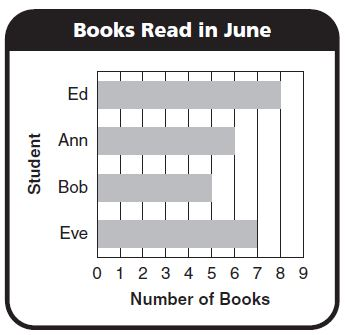Question 5.
How many more books did Ed read than Bob?
Options:
a. 2
b. 3
c. 4
d. 5

Explanation:

The bar graph shows that Ed read 8 books in June
Bob read 5 books in June
To know the number of books Ed read than Bob
We have to subtract the no. of books Bob read from Ed
= 8 – 5 = 3 books
So, the correct answer is option B

Question 6.
How many books in all did the four students read in June?
Options:
a. 22
b. 24
c. 26
d. 36

Explanation:

Bob read 5 books in June
Ed read 8 books in June
Eve read 7 books in June
Ann read 6 books in June
Total = 5 + 8 + 7 + 6 = 26 books
So, the answer is option C

### Multiply 1-Digit Numbers by Multiplies of 10 Page No 291

Find the product. Use base-ten blocks or draw a quick picture.

Question 1.
4 × 50 = 200Explanation:

First, multiply the ones
4 × o ones = 0
Next, multiply the tens
4 × 5 tens = 200

Question 2.
60 × 3 = _______

Explanation:

Mutliply the ones
3 × o ones = 0
Now multiply the tens
3 × 6 tens = 18 tens = 180

Question 3.
_______ = 60 × 5

Explanation:

Multiply the ones
5 × 0 ones = 0
Multiply the tens
5 × 6 tens = 30 tens
= 300

Find the product.

Question 4.
3 0
× 8
—–
_______

Explanation:

Multiply the ones
8 × 0 ones = 0
Multiply the tens
8 × 3 tens = 24 tens = 240

Question 5.
5 0
× 2
—–
_______

Explanation:

Multiply the ones
2 × 0 ones = 0
Multiply the tens
2 × 5 tens = 10 tens = 100

Question 6.
6 0
× 7
—–
_______

Explanation:

Multiply the ones
7 × 0 ones = 0
Multiply the tens
7 × 6 tens = 42 tens = 420

Question 7.
70
× 4
—–
_______

Explanation:

Multiply the ones
4× 0 ones = 0
Multiply the tens
4 × 7 tens = 28 tens = 280

Question 8.
6 × 90 = _______

Explanation:

Multiply the ones
6 × 0 ones = 0
Multiply the tens
6 × 9 tens = 54 tens = 540

Question 9.
9 × 70 = _______

Explanation:

Multiply the ones
9 × 0 ones = 0
Multiply the tens
9 × 7 tens = 63 tens = 630

Question 10.
8 × 90 = _______

Explanation:

Multiply the ones
8 × 0 ones = 0
Multiply the tens
8 × 9 tens = 72 tens = 720

Question 11.
_______ = 6 × 80

Explanation:

Multiply the ones
6 × 0 ones = 0
Multiply the tens
6 × 8 tens = 48 tens = 480

Problem Solving

Question 12.
Each model car in a set costs $4. There are 30 different model cars in the set. How much would it cost to buy all the model cars in the set?$ _______

Answer: $120 Explanation: There are 30 different model cars in the set Each model car in a set costs$4
To find the cost to buy all the model cars in the set
30 × $4 =$120

Question 13.
Amanda exercises for 50 minutes each day. How many minutes will she exercise in 7 days?
minutes _______

Explanation:

Given,
Amanda exercises for 50 minutes each day
We have to find how many minutes will she exercise in 7 days
50 × 7 = 350 minutes

### Multiply 1-Digit Numbers by Multiplies of 10 Lesson Check Page No 292

Question 1.
Each shelf in one section of the library holds 30 books. There are 9 shelves in that section. How many books will these shelves hold?
Options:
a. 220
b. 260
c. 270
d. 280

Explanation:

Given,

Each shelf in one section of the library holds 30 books
There are 9 shelves in that section
30 × 9 = 3 tens × 9
= 27 tens = 270
Therefore the correct answer is option C

Question 2.
One can of juice mix makes 60 ounces of juice. How many ounces of juice can be made from 6 cans of juice mix?
Options:
a. 300 ounces
b. 360 ounces
c. 390 ounces
d. 600 ounces

Explanation:

Given,
One can of juice mix makes 60 ounces of juice
Number of ounces of juice can be made from 6 cans of juice mix
60 ounces × 6 = 6 tens × 6
= 36 tens = 360 ounces
Thus the answer is option B

Spiral Review

Question 3.
Sue bought 7 cans of tennis balls. There are 3 balls in each can. How many balls did Sue buy?
Options:
a. 10
b. 21
c. 28
d. 37

Explanation:

Sue bought 7 cans of tennis balls
There are 3 balls in each can
To know the number of balls Sue buy
We have multiply number of cans and number of balls
= 7 × 3 = 21 balls

Question 4.
Which is an example of the Commutative Property of Multiplication?
Options:
a. 3 + 4 = 4 + 3
b. 5 × 0 = 0
c. 1 × 7 = 7
d. 3 × 4 = 4 × 3

Answer: 3 × 4 = 4 × 3

Explanation:

According to the commutative property of multiplication, changing the order of the numbers we are multiplying, does not change the product.
a × b = b × a
3 × 4 = 4 × 3
Option D is the correct example of the Commutative property

Question 5.
Lyn drew this bar model to solve a problem. Which operation should she use to find the unknown number?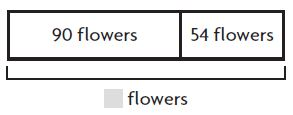Options:
b. division
c. multiplication
d. subtraction

Explanation:

In order to know the unknown number we have to add both the number of flowers
90 + 54 = 144

Question 6.
Joe drew this bar model to find the unknown number of balls. Which is the correct answer?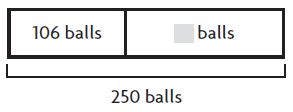Options:
a. 356
b. 256
c. 144
d. 124

Explanation:

Given that
Joe drew this bar model to find the unknown number of balls
106 balls + ___ = 250 balls
let the unknown number be x
106 + x = 250
x = 250 – 106
x = 144 balls

### Review/Test Page No 293

Question 1.
The camping club wants to rent rafts. Each raft can hold 8 people. Which equation could be used to find how many rafts are needed for 32 people?
Options:
a. 8 × 32 = ■
b. 32 × ■ = 8
c. ■ × 8 = 32
d. 32 × 8 = ■

Answer: ■ × 8 = 32

Explanation:

Each raft can hold 8 people
■ represents raft that can hold 32 people
Now we have to multiple number of people with rafts for 32 people
■ × 8 = 32
■ = 32/8
■ = 4
Therefore 4 rafts are needed for 32 people

Question 2.
Select the equations that show the Distributive Property. Mark all that apply.
Options:
a. 8 × 20 = 8 × (10 + 10)
b. 5 × 60 = 5 × (20 + 40)
c. 30 × 6 = 6 × 30
d. 9 × (4 + 3) = 9 × 7

Explanation:

The sum of two numbers times a third number is equal to the sum of each addend times the third number.
The above 3 options satisfy the law of Distributive Property

Question 3.
Choose the number from the box that makes the sentence true.
A library has 48 shelves of fiction books. There are 6 shelves in each cabinet.
There are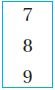cabinets of fiction books in the library.
_________

Explanation:

Let x represents cabinets of fiction in the library
x × 6 = 48
x = 48/6
x = 8

### Review/Test Page No 294

Question 4.
For numbers 4a–4d, choose True or False for each equation.
a. 5 × (4 + 4) = 8 × 5
i. True
ii. False

Explanation:

The above question satisfies the distributive property
5 × (4 + 4) = 5 × 8 = 40
8 × 5 = 40
Therefore LHS = RHS
So, the equation is true

Question 4.
b. 8 × (3 + 3) = 8 × 5
i. True
ii. False

Explanation:

8 × (3 + 3) = 8 × 6 = 48
8 × 5 = 40
So, the equation is false

Question 4.
c. (3 × 5) + (5 × 5) = 8 × 5
i. True
ii. False

Explanation:

(3 × 5) + (5 × 5) = 8 × 5
The above question satisfies the distributive property
8 × 5 = 40
(3 × 5) + (5 × 5) = 15 + 25 = 40
Both LHS and RHS are equal

Question 4.
d. (3 × 2) + (8 × 3) = 8 × 5
i. True
ii. False

Explanation:

(3 × 2) + (8 × 3)
3 × 2 = 6; 8 × 3 = 24
= (3 × 2) + (8 × 3) = 6 + 24
But it is given as 8 × 5

Question 5.
Alya planted 30 trays of flowers. Each tray held 8 flowers. Javon planted 230 flowers. Did Alya plant more flowers than Javon, the same number of flowers as Javon, or fewer flowers than Javon?
Options:
a. She planted more flowers than Javon.
b. She planted the exact same number of flowers as Javon.
c. She planted fewer flowers than Javon. 6.

Answer: She planted more flowers than Javon.

Explanation:

Given that, Alya planted 30 trays of flowers and each tray held 8 flowers
30 × 8 = 240 flowers
So, Alya planted 240 flowers
Javon planted 230 flowers
To know whether Alya plant more flowers than Javon, the same number of flowers as Javon, or fewer flowers than Javon
We have to subtract Number of flowers planted by Javon from Alya
240 – 230 = 10
So, Alya planted more flowers than Javon

Question 6.
For numbers, 6a–6d, choose Yes or No to show whether the unknown number is 6.

a. 4 × ■ = 32

i. Yes
ii. No

Explanation:

■ = 6
Now we have to substitute ■ = 6 in the above question
4 × 6 = 24

b. ■ × 6 = 36

i. Yes
ii. No

Explanation:

■ = 6
6 × 6 = 36
It satisfies the above equation

c. 8 × ■ = 49

i. Yes
ii. No

Explanation:

■ = 6
8 × 6 = 48 but not 49

d. ■ × 30 = 180

i. Yes
ii. No

Explanation:

■ = 6
6 × 30 = 180
It satisfies the above equation

Question 7.

Each train can carry 20 cars. Use the number line to find how many cars 6 trains can carry.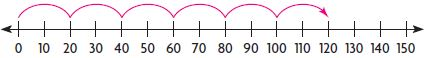Explanation:

Each train can carry 20 cars
6 trains can carry x cars
x × 1 = 20 × 6
x = 120 cars

### Review/Test Page No 295

Question 8.
Samantha made this multiplication model. Complete the equation that represents the model._____ × _____ = _____
Type below:
__________

Explanation:

Each vertical bar represents a ten. Each group of 3 vertical bars represents 30
We have 3 groups of 30, therefore the equation that represents the model is
3 × 30 = 90 models

Question 9.
A printer prints newsletters for many groups every month. Which group uses the greatest number of pieces of paper?__________

Explanation:

From the above table, we can say that Book Lovers Club group uses the greatest number of pieces of paper

Question 10.
A store has 30 boxes of melons. Each box holds 4 bags. Each bag holds 2 melons. What is the total number of melons in the store?
__________ melons

Explanation:

Given,
A store has 30 boxes of melons
Each box holds 4 bags
Each bag holds 2 melons
1 box holds 4 bags
30 × 4 = 120 bags
1 bag = 2 melons
120 bags = x
x = 120 × 2
x = 240 melons
Therefore total number of melons in the store = 240 melons

Question 11.
Heather’s puppy weighs 23 pounds. He has been gaining 3 pounds every month as he grows. If this pattern continues, how much will the puppy weigh 5 months
from now?
__________ pounds

Explanation:

Heather’s puppy weighs 23 pounds
He has been gaining 3 pounds every month as he grows
To find the weigh of the puppy after 5 months
If puppy weights 3 pounds in 1 month
For 5 months it weighs = 3 × 5 = 15 pounds
Actual of Heather’s puppy + puppy weight after 5 months
= 23 + 15 = 38 pounds

### Review/Test Page No 296

Question 12.
Tim describes a pattern. He says the pattern shown in the table is “Add 3.” Is Tim correct? Explain how you know.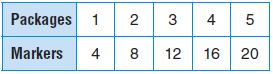Type below:
__________

No, Tim is not correct
The pattern works for the first pair of numbers 1 + 3 = 4
But it doesn’t work for any of the other pairs. The pattern should be Multiply the number of packages by 4

Question 13.
This shows a part of a multiplication table. Find the missing numbers. Explain how you found the numbers.Type below:
__________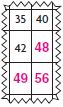Explanation:

The only numbers that have a product of 35 are 5 and 7. The only numbers that have a product of 40 are 5 and 8.
This tells us that 5 is the number for the row. That means 6 is the next row down, and 7 is the row after that. The factors of 7 and 8 are the columns, so we can multiply to find the missing numbers

Question 14.
Describe a pattern for this table.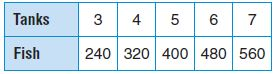Pattern: _____
How would the table change if the pattern was “Multiply the number of tanks by 8”? Explain.
Type below:
__________

Answer: Multiply the number of tanks by 80
The table would change by taking a zero off each number of fish in the second row because you would be multiplying by ones, not tens.

### Review/Test Page No 297

Question 15.
Devon has 80 books to pack in boxes. She packs 20 books in each box. How many boxes does she need?
Write an equation using the letter n to stand for the unknown factor. Explain how to find the unknown factor.
Type below:
__________

Answer: n × 20 = 80
We can draw an array of 80 squares with 20 squares in each row.
There are 4 rows, so n = 4. Devon needs 4 boxes.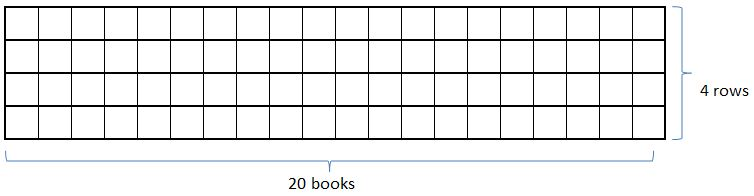Question 16.
The bookstore has 6 shelves of books about animals. There are 30 books on each shelf. How many books about animals does the bookstore have?
Shade squares to make a diagram to show how you can use the Distributive Property to find the number of books about animals in the bookstore.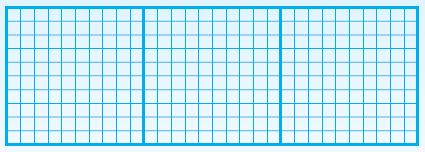__________ animal books

6 × (10 + 10 + 10) = (6 × 10) + (6 × 10) + (6 × 10)
= 60 + 60 + 60
= 180 animal books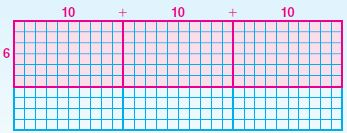### Review/Test Page No 298

Question 17.
Cody saves all his nickels. Today he is getting them out of his piggy bank and wrapping them to take to the bank. He finds he has 360 nickels. It takes 40 nickels to fill each paper wrapper and make a roll. How many wrappers does he need?
Part A
Write an equation using n for the unknown number. Find the number of wrappers needed.
______ × ______ = ______

Answer: n × 40 = 360

Explanation:

n represents no. of wrappers need
Each paper wrapper needs 40 nickels
Cody has 360 nickels
n × 40 = 360

Question 17.
Part B
Explain how you solved this problem and how you know your answer is correct.
Type below:
__________

Explanation:

Given that Cony has 360 nickels
Each paper wrapper needs 40 nickels
Let n be the number of wrappers needed
That means n × 40 = 360
n = 360/40
n = 9
So, n wrappers are needed to make the rolls

Question 18.
Ruben is collecting cans for the recycling contest at school. He makes two plans to try to collect the most cans.
Plan A: Collect 20 cans each week for 9 weeks.
Plan B: Collect 30 cans each week for 7 weeks.
Part A
Which plan should Ruben choose?
__________

Collect 30 cans each week for 7 weeks.

Question 18.
Part B